# Expanded Notation Method for Division

Instructor: Nola Bridgens

Nola has taught elementary school and tutored for four years. She has a bachelor's degree in Elementary Education, a master's degree in Marketing, and is a certified teacher.

In this lesson, you will learn the pattern of dividing, multiplying, and subtracting that makes up the expanded notation method for division. You will then use this method to solve some problems.

## The Long Way

Picture yourself blowing up a balloon. What happens when you blow air in? It expands - it gets bigger. Now look at this: 300 + 40 + 7. You remember what this is, right? It is the number 347 written in expanded notation - just a bigger form of the same number. Did you know that there is an expanded notation method for division which uses expanded notation to solve long division problems? Let's see how!

## Dividing With Expanded Notation

As we just reviewed, expanded notation keeps a number's place value and helps us visualize exactly what the numbers represent. Solving long division problems with expanded notation is just a repeated series of steps that involves dividing, multiplying, and subtracting. As with all division problems, it is important that you know your multiplication facts very well, as it is a major part of the long division process.

Remember this pattern: 'Divide, Multiply, Subtract' (÷) (x) (-) and you will do just fine. Let's think about this pattern as we go through the first example.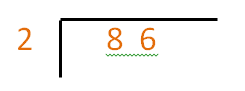Step 1: (÷)

How many times can 2 go into 86?

We know that 2 can go into 8 four times, so it can go into 86 at least 40 times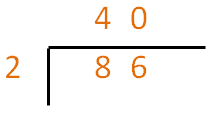Step 2: (x)

Multiply the answer (40) by the divisor (2) and write the answer under the dividend.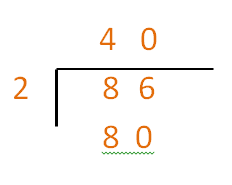Step 3: (-)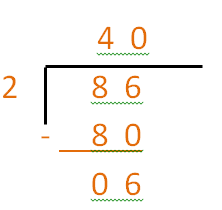Now we repeat the (÷) (x) (-) pattern.

Step 4: (÷)

How many times can 2 go into 6?

6 ÷ 2 = 3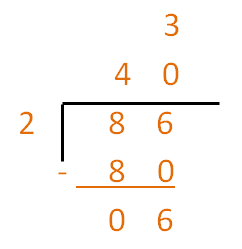Step 5: (x)

Multiply the answer (3) by the divisor (2) and write the answer under the second number of the dividend.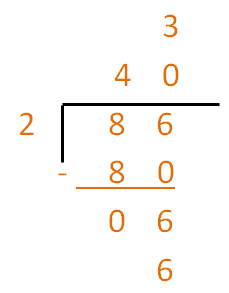Step 6: (-)

Subtract your answer (6) from the second number of the dividend (6) and the answer is 0.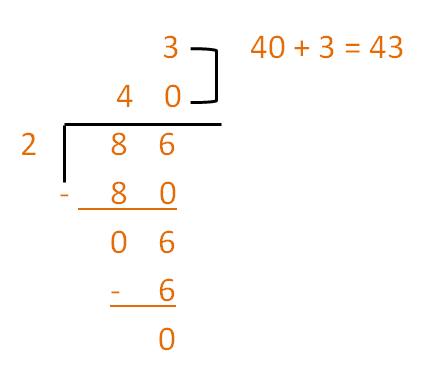When you have no more numbers to divide into, you are finished. You then find the final answer by adding the numbers above the division sign. In this problem, you add 40 + 3 and the answer is 43.

## Three Digit Dividend

Let's take our 'Divide, Multiply, Subtract' pattern and try a division problem with a three digit dividend.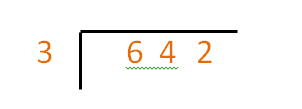Step 1: (÷)

How many times can 3 go into 600?

We know 3 goes into 6 twice, so it will go into 642 at least 200 times

Step 2: (x)

Multiply the answer (200) by the divisor (3) and write the answer under the dividend.

Step 3: (-)

Now we repeat the 'Divide, Multiply, Subtract' pattern.

Step 4: (÷)

How many times can 3 go into 42?

To unlock this lesson you must be a Study.com Member.

### Register to view this lesson

Are you a student or a teacher?

#### See for yourself why 30 million people use Study.com

##### Become a Study.com member and start learning now.
Back
What teachers are saying about Study.com

### Earning College Credit

Did you know… We have over 200 college courses that prepare you to earn credit by exam that is accepted by over 1,500 colleges and universities. You can test out of the first two years of college and save thousands off your degree. Anyone can earn credit-by-exam regardless of age or education level.